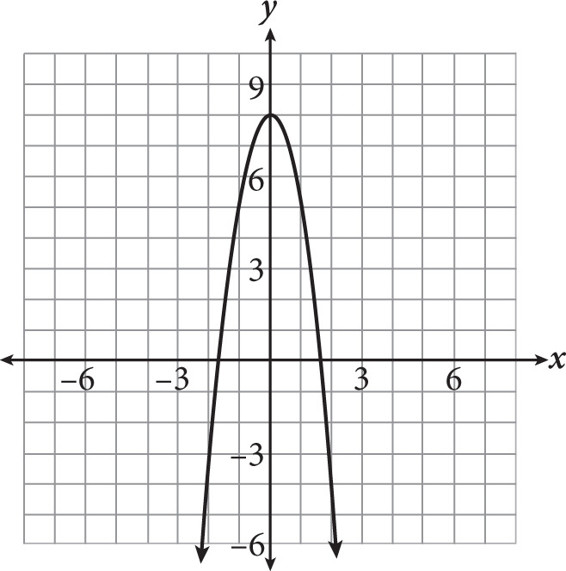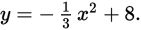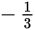# SAT Math Multiple Choice Question 292: Answer and Explanation

### Test Information

Question: 292

7.Vadim graphs the equation y = –3x2 + 8, which is shown in the figure above. He realizes, however, that he miscalculated and should have graphedHow will this affect his graph?

• A. It will change the y-intercept.
• B. It will make the parabola open in the opposite direction.
• C. It will make the parabola cross the x-axis closer to the origin.
• D. It will make the parabola cross the x-axis farther from the origin.

Getting to the Answer: Changing the coefficient of x2 from -3 towill make the graph narrower or wider (in this case, wider), which means the only things that will change are the x-intercepts. This means you can eliminate A and B. To choose between C and (D), recall that fraction coefficients (between 0 and 1) result in wider graphs, so the x-intercepts will spread out and therefore be farther from the origin.